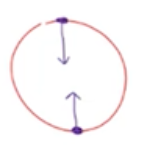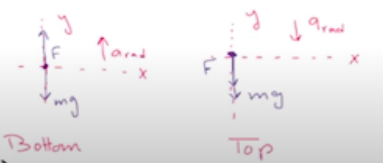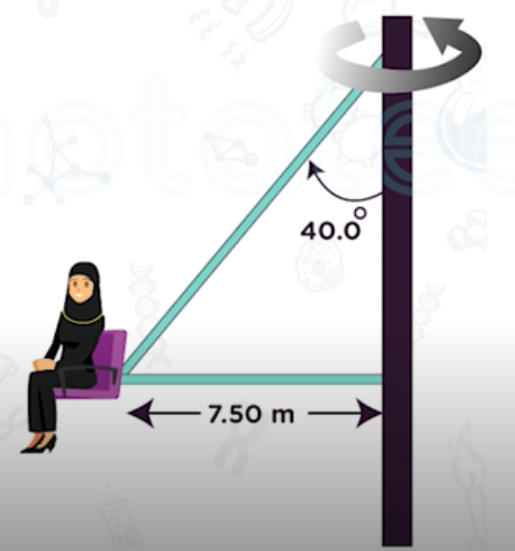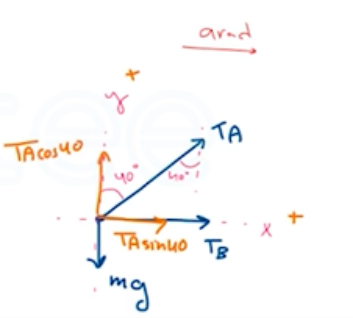Need Help?

Subscribe to Physics 1

###### \${selected_topic_name}
• Notes

$\begin{array}{l}{\text { A machine part con-sists of a thin } 40.0 \text { -cm-long bar }} \\ {\text { with small } 1.15-\mathrm{kg} \text { masses fas-tened by screws to its ends. }} \\ {\text { The screws can support a maximum }}\end{array}$

$\begin{array}{l}{\text { force of } 75.0 \mathrm{N} \text { without pulling out. This bar rotates about an axis }} \\ {\text { perpendicular to it at its center. (a) As the bar is turning at a constant }} \\ {\text { rate on a horizontal, frictionless surface, what is the maximum }} \\ {\text { speed the masses can have without pulling out the screws? (b) }} \\ {\text { Suppose the machine is redesigned so that the bar turns at a con- }}\end{array}$

$\begin{array}{l}{\text { stant rate in a vertical circle. Will one of the screws be more likely }} \\ {\text { to pull out when the mass is at the top of the circle or at the bot- }} \\ {\text { tom? Use a free-body diagram to see why. (c) Using the result of }} \\ {\text { part (b), what is the greatest speed the masses can have without }} \\ {\text { pulling a screw? }}\end{array}$$a_{rad}=\frac{v^{2}}{R}$

$R=0.2 \mathrm{m}$

$(a) a_{x}=a_{rad} \quad(b) a_ y=a_{r ad}$

$\text { (a) }\sum F_{x}=m a_{x} \rightarrow F=m a_{ra d}$

$\rightarrow F=m \frac{v^{2}}{R} \rightarrow v=\sqrt{\frac{F R}{m}}$

$V=\sqrt{\frac{75 * 0.2}{1.15}}=3.61 \mathrm{m} / \mathrm{s}$$\rightarrow \text { At The top }\sum F_ y= ma_y$

$F=m a_{rad}-m g$

$\rightarrow \text { At the bottom } \sum F_y=ma_y$

$F=m a_{rad}+m g$

$F=m a_ {r a d}+mg \rightarrow F=m(a_{rad}+g)$

$\frac{F}{m}=a_{rad}+g \rightarrow a_{rad}=\frac{F}{m}-g \Rightarrow \frac{\left(V^{2}\right)}{R}=\frac{F}{m}-g$

$∴ V=\sqrt{R\left(\frac{F}{m}-g\right)}=\sqrt{0.2(\frac{75}{1.15}-9.8)}=3.35 \mathrm{m/s}$

$\begin{array}{l}{\text { In another version of the "Giant Swing" (see Exer-cise 5.46), the seat is connected }} \\ {\text { to two cables as shown in Fig. }} \\ {\text { E5.47, one of which is horizon-tal. The seat swings in a hori-zontal circle at a rate of 32.0 }} \\ {\text { rpm (rev/min). If the seat weighs 255 N and an } 825-\mathrm{N} \text { per-son is sitting in it, find the ten- }} \\ {\text { sion in each cable. }}\end{array}$$R=7.5 \mathrm{m}$

$w_{s}=255 \mathrm{N}, \quad {w}_{\mathrm{p}}=825 \mathrm{N}$

$w_{t o t al}=255+825$

$m_{t o t al}=\frac{w}{g}=\frac{255+825}{9.8}=110.2 \mathrm{kg}$

$32 \text { rev/min }=0.5333 \mathrm{rev} / \mathrm{s}$

$T=\frac{1}{0.5333}=1.875 \mathrm{s}$

$\sum F_ y=m a_ y \rightarrow T_{A} \cos 4{0}-m g=0 \rightarrow T_{A}=\frac{m g}{ \cos 4{0}}=1410N$

$\sum F_{x}=m a_ x \rightarrow T_{A} \sin 40+T_{13}=ma_{rad}\rightarrow T_{13}=ma_{rad}-T_A \sin 40$

$\rightarrow T_{B}=m\left(\frac{4 \pi^{2} R}{T^{2}}\right)-T_{A} \sin 40=110.2\left(\frac{4 \pi^{2}(7.5)}{(1.875)^{2}}\right)-(1410) \sin 4{0}=8370N$